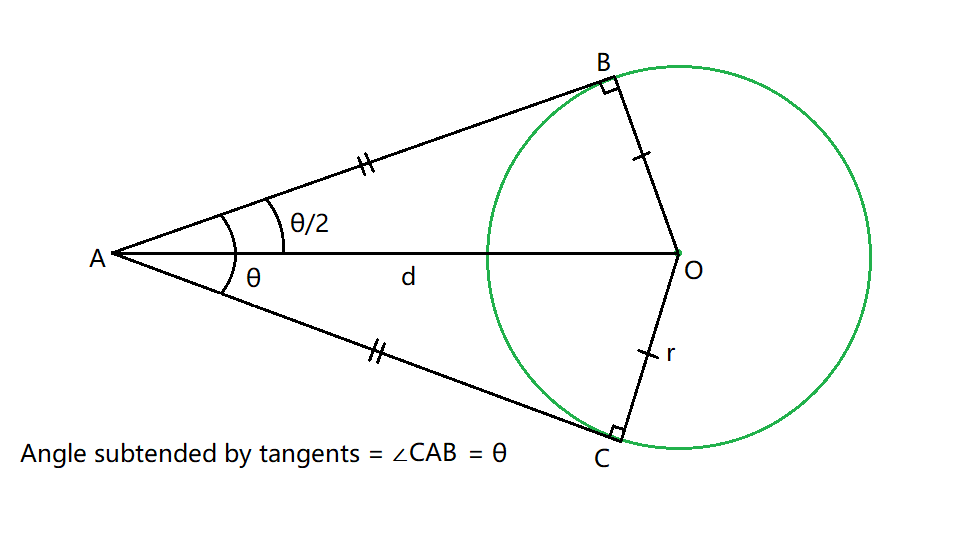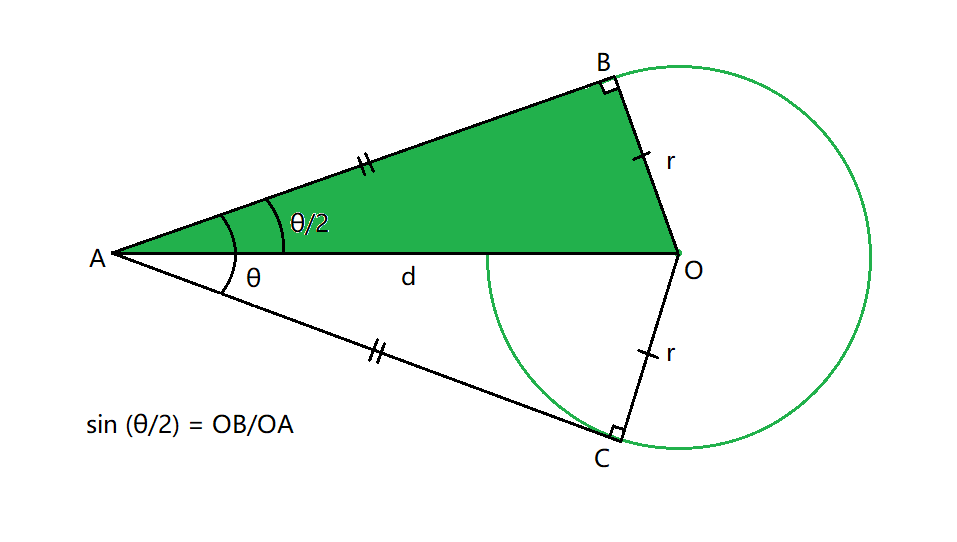GFG App
Open AppBrowser
Continue

# Find the angle between tangents drawn from a given external point to a Circle

Given a positive integer R representing the radius of the circle and the center of the circle (X1, Y1) and another point (X2, Y2) in the cartesian plane, the task is to find the angle between the pair of tangents drawn from the point (X2, Y2) to the circle.Examples:

Input: R = 6, (X1, Y1) = (5, 1), (X2, Y2) = (6, 9)
Output: 96.1851

Input: R = 4, (X1, Y1) = (7, 12), (X2, Y2) = (3, 4)
Output: 53.1317

Approach: The given problem can be solved based on the following observations:

• The radius makes an angle of 90 degrees with the tangent at the point of contact of the tangent and circle. Also, the angle subtended by the pair of tangents (θ) is bisected by the line joining the center of the circle and the exterior point.
• Therefore, the distance between the center and the exterior point can be calculated using the distance formula as:

Distance =Now, consider d as the distance between the two given points, then In the right-angled triangle OAB,

=>=>=>=>Therefore, using the above formula, the angle between the pair of tangents drawn from the point (X2, Y2) to the circle can be calculated.

Below is the implementation of the above approach:

## C++

 // C++ program for the above approach   #include  #include  using namespace std;   // Function to find the distance between // center and the exterior point double point_distance(int x1, int y1,                       int x2, int y2) {     // Find the difference between     // the x and y coordinates     int p = (x2 - x1);     int q = (y2 - y1);       // Using the distance formula     double distance = sqrt(p * p                            + q * q);       return distance; }   // Function to find the angle between // the pair of tangents drawn from the // point (X2, Y2) to the circle. void tangentAngle(int x1, int y1,                   int x2, int y2,                   double radius) {     // Calculate the distance between     // the center and exterior point     double distance = point_distance(         x1, y1, x2, y2);       // Invalid Case     if (radius / distance > 1         || radius / distance < -1) {         cout << -1;     }       // Find the angle using the formula     double result         = 2 * asin(radius / distance) * 180           / 3.1415;       // Print the resultant angle     cout << result << " degrees"; }   // Driver Code int main() {     int radius = 4;     int x1 = 7, y1 = 12;     int x2 = 3, y2 = 4;     tangentAngle(x1, y1, x2, y2, radius);       return 0; }

## Java

 // java program for the above approach import java.io.*; import java.lang.*; import java.util.*;   class GFG {     // Function to find the distance between // center and the exterior point static double point_distance(int x1, int y1,                       int x2, int y2) {     // Find the difference between     // the x and y coordinates     int p = (x2 - x1);     int q = (y2 - y1);        // Using the distance formula     double distance = Math.sqrt(p * p                            + q * q);        return distance; }    // Function to find the angle between // the pair of tangents drawn from the // point (X2, Y2) to the circle. static void tangentAngle(int x1, int y1,                   int x2, int y2,                   double radius) {         // Calculate the distance between     // the center and exterior point     double distance = point_distance(         x1, y1, x2, y2);        // Invalid Case     if (radius / distance > 1         || radius / distance < -1) {          System.out.println(-1);     }        // Find the angle using the formula     double result         = 2 * Math.asin(radius / distance) * 180           / 3.1415;        // Print the resultant angle     System.out.println(String.format("%.4f", result) + " degrees"); }         // Driver Code     public static void main(String[] args)     {       int radius = 4;     int x1 = 7, y1 = 12;     int x2 = 3, y2 = 4;     tangentAngle(x1, y1, x2, y2, radius);     } }   // This code is contributed by susmitakundugoaldanga.

## Python3

 # Python 3 program for the above approach import math   # Function to find the distance between # center and the exterior point def point_distance(x1, y1,                    x2,  y2):       # Find the difference between     # the x and y coordinates     p = (x2 - x1)     q = (y2 - y1)       # Using the distance formula     distance = math.sqrt(p * p                          + q * q)       return distance   # Function to find the angle between # the pair of tangents drawn from the # point (X2, Y2) to the circle. def tangentAngle(x1,  y1,                  x2,  y2,                  radius):       # Calculate the distance between     # the center and exterior point     distance = point_distance(         x1, y1, x2, y2)       # Invalid Case     if (radius / distance > 1             or radius / distance < -1):         print(-1)       # Find the angle using the formula     result = 2 * math.asin(radius / distance) * 180 / 3.1415       # Print the resultant angle     print(result, " degrees")   # Driver Code if __name__ == "__main__":       radius = 4     x1 = 7     y1 = 12     x2 = 3     y2 = 4     tangentAngle(x1, y1, x2, y2, radius)       # This code is contributed by ukasp.

## C#

 // C# program for the above approach using System;   class GFG{   // Function to find the distance between // center and the exterior point static double point_distance(int x1, int y1,                              int x2, int y2) {           // Find the difference between     // the x and y coordinates     int p = (x2 - x1);     int q = (y2 - y1);       // Using the distance formula     double distance = Math.Sqrt(p * p + q * q);       return distance; }   // Function to find the angle between // the pair of tangents drawn from the // point (X2, Y2) to the circle. static void tangentAngle(int x1, int y1, int x2,                           int y2, double radius) {       // Calculate the distance between     // the center and exterior point     double distance = point_distance(x1, y1, x2, y2);       // Invalid Case     if (radius / distance > 1 ||          radius / distance < -1)     {         Console.WriteLine(-1);     }       // Find the angle using the formula     double result = 2 * Math.Asin(                radius / distance) *                    180 / 3.1415;       // Print the resultant angle     Console.WriteLine(         String.Format("{0:0.0000}", result) +                        " degrees"); }   // Driver code static void Main() {     int radius = 4;     int x1 = 7, y1 = 12;     int x2 = 3, y2 = 4;           tangentAngle(x1, y1, x2, y2, radius); } }   // This code is contributed by abhinavjain194

## Javascript

 

Output:

53.1317 degrees

Time Complexity: O(logn) where n = (x2-x1)2+(y2-y1)2, because using inbuilt sqrt function
Auxiliary Space: O(1)

My Personal Notes arrow_drop_up